## 11.4.1 Discrete-Stage Information Spaces

Assume here that there are discrete stages. Let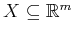be an-dimensional manifold for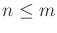called the state space.11.4 Let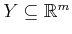be an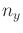-dimensional manifold for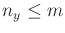called the observation space. For each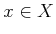, let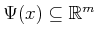be an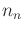-dimensional manifold for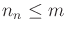called the set of nature sensing actions. The three kinds of sensors mappings,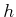, defined in Section 11.1.1 are possible, to yield either a state mapping,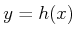, a state-nature mapping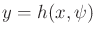, or a history-based,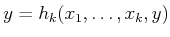. For the case of a state mapping, the preimages,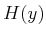, once again induce a partition of. Preimages can also be defined for state-action mappings, but they do not necessarily induce a partition of.

Many interesting sensing models can be formulated in continuous state spaces. Section 11.5.1 provides a kind of sensor catalog. There is once again the choice of nondeterministic or probabilistic uncertainty if nature sensing actions are used. If nondeterministic uncertainty is used, the expressions are the same as the discrete case. Probabilistic models are defined in terms of a probability density function,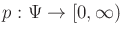,11.5 in which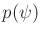denotes the continuous-time replacement for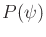. The model can also be expressed as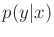, in that same manner that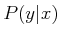was obtained for discrete state spaces.

The usual three choices exist for the initial conditions: 1) Either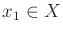is given; 2) a subset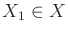is given; or 3) a probability density function,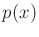, is given. The initial condition spaces in the last two cases can be enormous. For example, if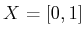and any subset is possible as an initial condition, then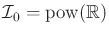, which has higher cardinality than. If any probability density function is possible, then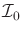is a space of functions.11.6

The I-space definitions from Section 11.1.2 remain the same, with the understanding that all of the variables are continuous. Thus, (11.17) and (11.19) serve as the definitions of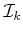and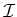. Let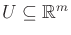be an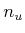-dimensional manifold for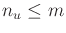. For eachand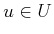, let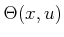be an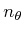-dimensional manifold for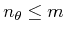. A discrete-stage I-space planning problem over continuous state spaces can be easily formulated by replacing each discrete variable in Formulation 11.1 by its continuous counterpart that uses the same notation. Therefore, the full formulation is not given.

Steven M LaValle 2020-08-14